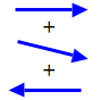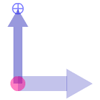You may also likeArrow Arithmetic 1

The first part of an investigation into how to represent numbers using geometric transformations that ultimately leads us to discover numbers not on the number line.Arrow Arithmetic 2

Introduces the idea of a twizzle to represent number and asks how one can use this representation to add and subtract geometrically.Twizzle Arithmetic

Arrow arithmetic, but with a twist.

Arrow Arithmetic 3

Age 14 to 16 Challenge Level:

This problem follows on from Arrow Arithmetic 2 .

Things start to get interesting when we try to multiply twizzles together. In the following animation, the grey twizzle is the product of the blue and green twizzles. You can try several multiplications by unlocking the green and blue twizzles and changing their values.

How can you construct the grey twizzle by transforming the green and blue twizzles?

Full Screen Version
If you can see this message Flash may not be working in your browser
Please see http://nrich.maths.org/techhelp/#flash to enable it.

If you switch the unit arrow and the number arrow in a twizzle, how does its value change?

In the following animation, the grey twizzle is the blue twizzle divided by the green twizzle. How could you construct a quotient twizzle like this grey one with the right value?
Full Screen Version
If you can see this message Flash may not be working in your browser
Please see http://nrich.maths.org/techhelp/#flash to enable it.